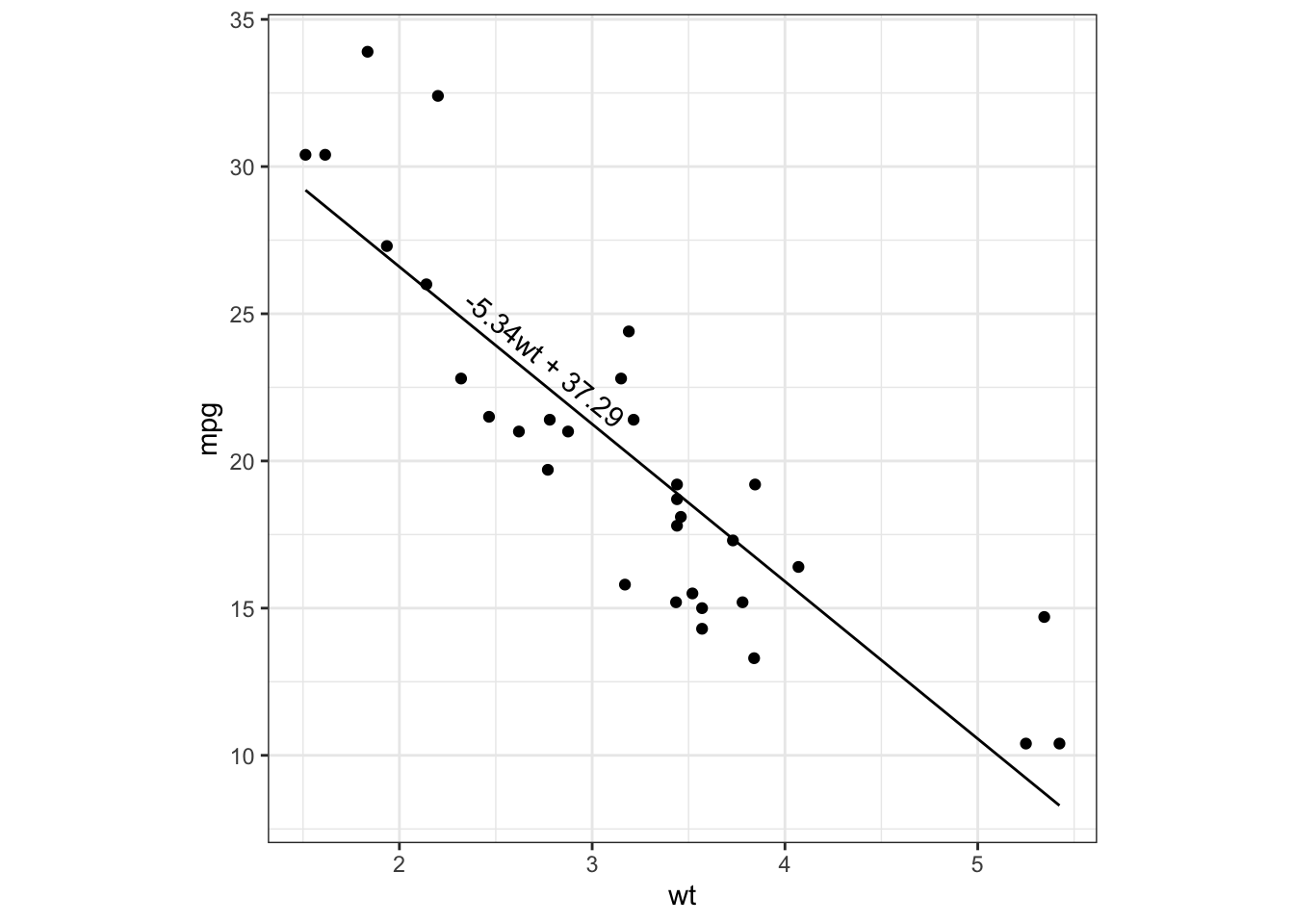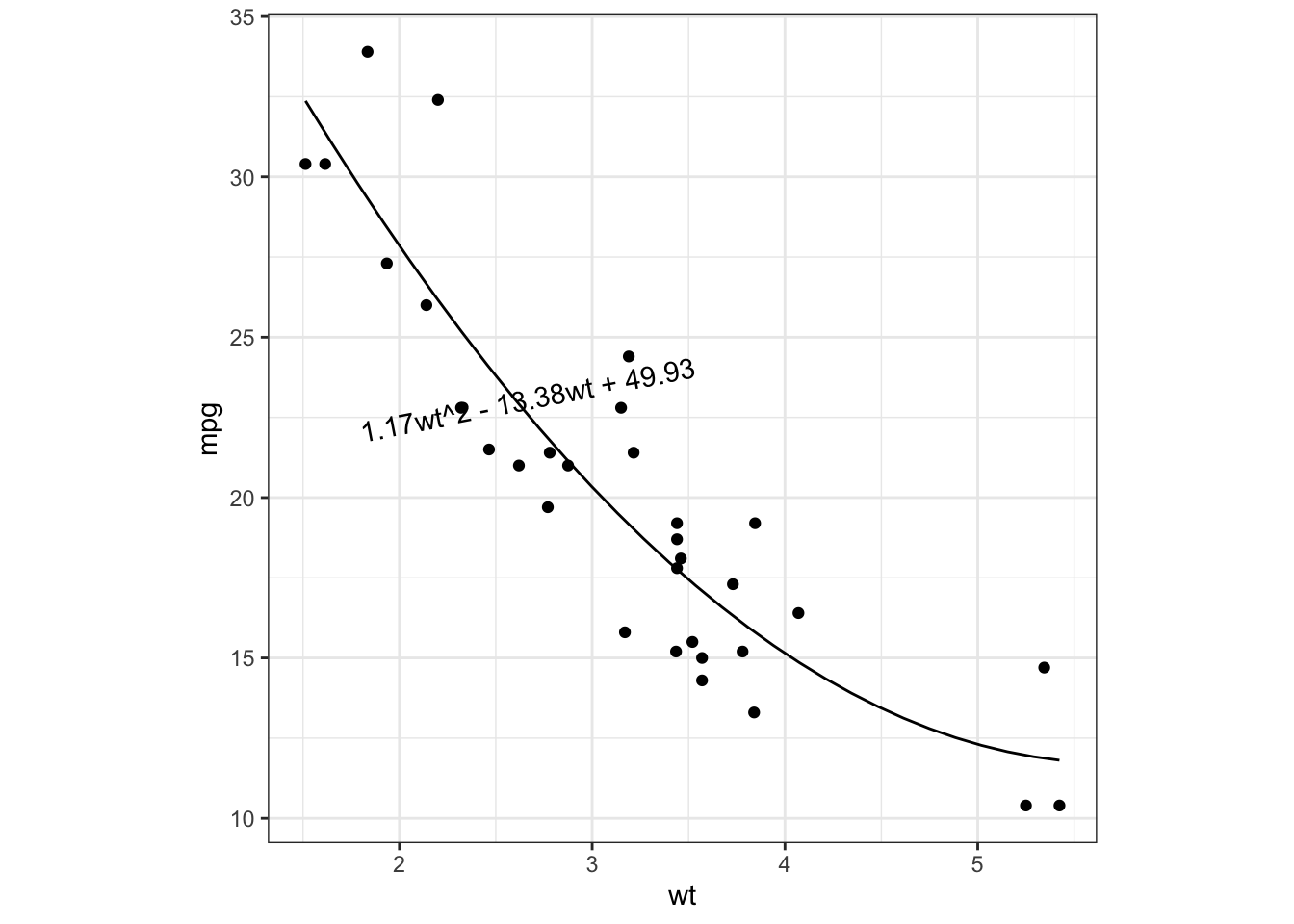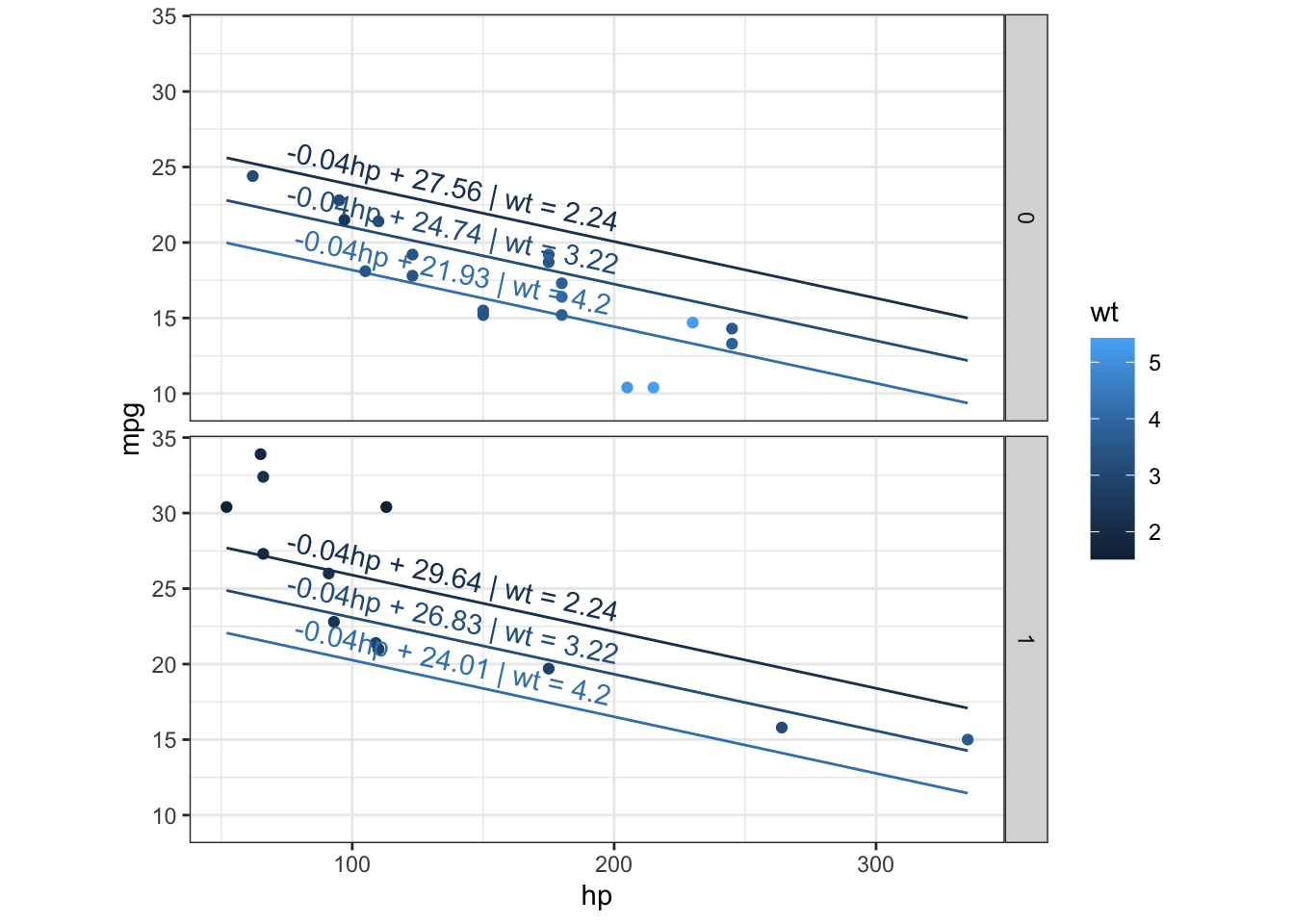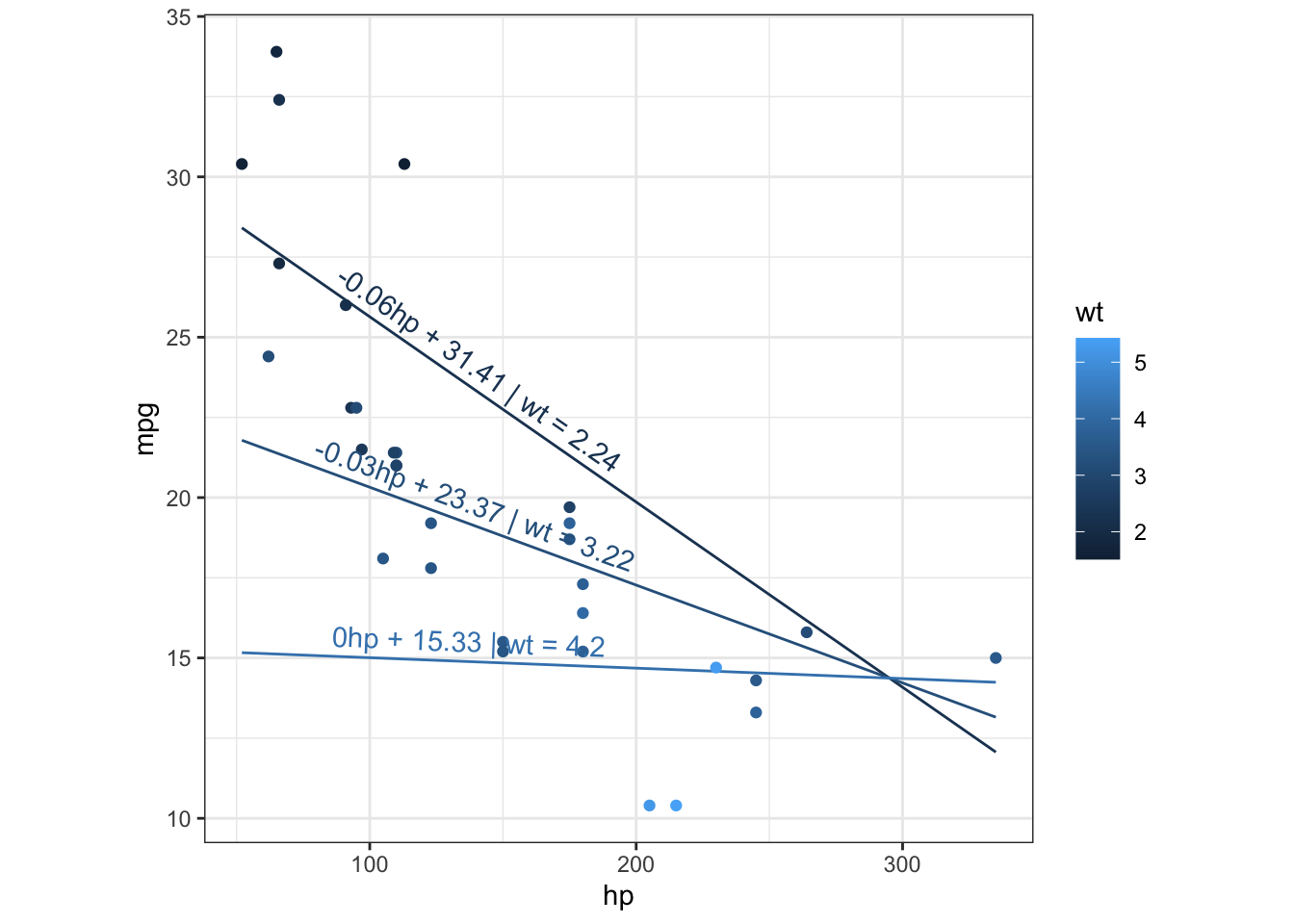메뉴 건너뛰기

# 회귀모형 자동해석

2020.03.08 22:25

조회 수:87

``안녕하세요? 문건웅입니다. 이번에 웹R프로그램을 대대적으로 다시 짜고 있습니다. 프로그램을 손보는 김에 우선 회귀모형에 대한 설명을 해주는 함수를 만들어 보았습니다. 제가 영어도 짧고 통계가 전공이 아니어서 오류가 있을까 걱정됩니다. 한번 보시고 평가해주시면 고맙겠습니다. ``

``````library(webrSub)
library(predict3d)``````

## Simple linear regression

``````fit=lm(mpg~wt,data=mtcars)
ggPredict(fit)````````````cat(result=explain(fit))
The predictor variable 'wt' accounts for 75.28 % of the total variation of your dependent variable 'mpg'. Your R^2 is quite good. The coefficient for 'wt' is smaller than zero. This means that the value of your dependent variable 'mpg' decreases by 5.34 for any increase of 1 in your independent variable 'wt'. The coefficient is  significantly different from zero(p< 0.001). The predicted value of dependent variable 'mpg' is calculated by the equation: '37.29 - 5.34*wt'.``````

## Polynomial regression

``````fit=lm(mpg~wt+I(wt^2),data=mtcars)
ggPredict(fit)````````````cat(explain(fit))
The predictor variables 'wt','I(wt^2)' account for 81.91 % of the total variation of your dependent variable 'mpg'. Your R^2 is quite good. The coefficient for 'wt' is  significantly different from zero(p< 0.001) The coefficient for 'I(wt^2)' is  significantly different from zero(p =0.003) The predicted value of dependent variable 'mpg' is calculated by the equation: '49.93 - 13.38*wt + 1.17*I(wt^2)'.``````

## Multiple linear regression

``````fit=lm(mpg~hp+wt+am,data=mtcars)
ggPredict(fit)````````````cat(explain(fit))
The predictor variables 'hp','wt','am' account for 83.99 % of the total variation of your dependent variable 'mpg'. Your R^2 is quite good. The coefficient for 'hp' is smaller than zero. This means that the value of your dependent variable 'mpg' decreases by 0.037 for any increase of 1 in your independent variable 'hp', if 'wt','am' remain(s) constant. The coefficient is  significantly different from zero(p< 0.001), controlling for the other variable(s). The coefficient for 'wt' is smaller than zero. This means that the value of your dependent variable 'mpg' decreases by 2.88 for any increase of 1 in your independent variable 'wt', if 'hp','am' remain(s) constant. The coefficient is  significantly different from zero(p =0.004), controlling for the other variable(s). The coefficient for 'am' is larger than zero. This means that the value of your dependent variable 'mpg' increases by 2.08 for any increase of 1 in your independent variable 'am', if 'hp','wt' remain(s) constant. The coefficient is  not significantly different from zero(p =0.141) suggesting that 'am' and 'mpg' are not linearly related when controlling for other predictor variable(s). The predicted value of dependent variable 'mpg' is calculated by the equation: '34.00 - 0.04*hp - 2.88*wt + 2.08*am'.``````

## Multiple linear regression with interaction

``````fit=lm(mpg~hp*wt,data=mtcars)
ggPredict(fit)````````````cat(explain(fit))
The predictor variables 'hp','wt' and the interaction 'hp:wt' account for 88.48 % of the total variation of your dependent variable 'mpg'. Your R^2 is quite good. The interaction between 'hp' and 'wt' is significant. This means that the relationship between one predictor and the response variable depends on the level of the other variable(s). Here it means that the relationship between 'mpg' and 'hp' varies by 'wt'. The predicted value of dependent variable 'mpg' is calculated by the equation: '49.81 - 0.12*hp - 8.22*wt + 0.03*hp*wt' which simplifies to '49.81 - 8.22*wt + (-0.12 + 0.03*wt)*hp'. If 'wt' is 2.24, the value of your dependent variable 'mpg' decreases by 0.058 for any increase of 1 in your independent variable 'hp' If 'wt' is 3.22, the value of your dependent variable 'mpg' decreases by 0.031 for any increase of 1 in your independent variable 'hp' If 'wt' is 4.2, the value of your dependent variable 'mpg' decreases by 0.0033 for any increase of 1 in your independent variable 'hp'``````
번호 제목 글쓴이 날짜 조회 수
199 안녕하세요. 회귀식에 관해 문의드립니다.2020.03.24 8
198 교수님 오랜만입니다!2020.03.15 2
197 정회원 서버 프로그램 오류 관련 문의드립니다.2020.03.15 3
» 회귀모형 자동해석 2020.03.08 87
195 답변 부탁드리고 감사드립니다.2020.03.03 63
194 부탁드립니다2020.03.02 4
193 rstudio 계정 문의 드립니다.2020.02.17 2
192 문의드립니다.2020.02.12 61
191 문의드립니다.2020.02.10 3
190 정회원 서버에서 데이터를 업로드하였는데 변수선택이 안됩니다.2020.01.15 3
189 정회원 서버 접속이 안됩니다..2020.01.10 1
188 서버접속오류2020.01.06 2
187 정회원 서버 비밀번호를 변경하고싶습니다.2019.11.01 4
186 기존 정회원입니다2019.10.09 3
185 정회원 신청2019.09.26 6
184 rstudio 사이트에 연결할 수 없음 문제  2019.09.10 8908
183 정회원 서버가 안열립니다.2019.09.03 3
182 정회원 서버 접속이 안됩니다.2019.08.31 2
181 [정회원 서버 접속 불가]2019.08.27 3
180 그래프 출력 오류  2019.07.17 195